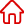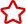﻿ php一種每天僅第一次啟動時執行的方法的實現_關於PHP編程設為首頁 加入收藏

C語言|JAVA編程
Python編程

ASP編程|PHP編程
JSP編程

MYSQL數據庫|SqlServer數據庫
Oracle數據庫|DB2數據庫
程式師世界 >> 編程語言 >> 網頁編程 >> PHP編程 >> 關於PHP編程 >> php一種每天僅第一次啟動時執行的方法的實現

# php一種每天僅第一次啟動時執行的方法的實現

//自動數據轉移
function turnMessage(){
set_time_limit(0);
ignore_user_abort(true);
global \$gzhuan,\$guser,\$hfdate1;
if('1'==\$gzhuan){
\$now = time();
\$time = date('Y-m-d',\$now);
\$file = 'include/time.txt';
\$f = fopen(\$file,'r+');
fclose(\$f);
if(\$t!=\$time){
\$timeok = date('Y-m-d',\$now-\$hfdate1*86400);
\$user = \$this->tmp_mod->getOneUser(\$guser);
if(\$user){
\$list = \$this->tmp_mod->getGuoqi(\$timeok);
if(\$list){
\$ymd = date('Y-m-d H:i:s',\$now);
\$d['hfzt'] = 1;
\$d['YyTime'] = \$time;
\$d['zhuanru'] = 1;
foreach(\$list as \$key=>\$val){
\$data['pro_id'] = \$val['ID'];
\$data['content'] = '系統自動轉入本數據，原預約時間為:'.\$val['YyTime'];
\$data['dates'] = \$ymd;
\$data['type'] = 0;
\$rs2 = \$this->tmp_mod->editProducts(\$val['ID'],\$d);
}
if(\$rs&&\$rs2){
parent::innerLog('自動轉移數據','1');
\$f = fopen(\$file,'w+');
fwrite(\$f,\$time);
fclose(\$f);
}else{
parent::innerLog('自動轉移數據','0');
}
}
}
}
}
unset(\$gzhuan,\$guser,\$hfdate1);
exit;
}

1. 上一頁:
2. 下一頁: